# C Pointers

Pointers are powerful features of C and C++ programming. Before we learn pointers, let's learn about addresses in C programming.

If you have a variable var in your program, `&var` will give you its address in the memory.

We have used address numerous times while using the `scanf()` function.

``scanf("%d", &var);``

Here, the value entered by the user is stored in the address of var variable. Let's take a working example.

``````#include <stdio.h>
int main()
{
int var = 5;
printf("var: %d\n", var);

// Notice the use of & before var
return 0;
}
``````

Output

```var: 5

Note: You will probably get a different address when you run the above code.

## C Pointers

Pointers (pointer variables) are special variables that are used to store addresses rather than values.

### Pointer Syntax

Here is how we can declare pointers.

``int* p;``

Here, we have declared a pointer p of `int` type.

You can also declare pointers in these ways.

``````int *p1;
int * p2;
``````

Let's take another example of declaring pointers.

``int* p1, p2;``

Here, we have declared a pointer p1 and a normal variable p2.

Let's take an example.

``````int* pc, c;
c = 5;
pc = &c;
``````

Here, 5 is assigned to the c variable. And, the address of c is assigned to the pc pointer.

### Get Value of Thing Pointed by Pointers

To get the value of the thing pointed by the pointers, we use the `*` operator. For example:

``````int* pc, c;
c = 5;
pc = &c;
printf("%d", *pc);   // Output: 5``````

Here, the address of `c` is assigned to the pc pointer. To get the value stored in that address, we used *pc.

Note: In the above example, pc is a pointer, not `*pc`. You cannot and should not do something like `*pc = &c`;

By the way, `*` is called the dereference operator (when working with pointers). It operates on a pointer and gives the value stored in that pointer.

### Changing Value Pointed by Pointers

Let's take an example.

``````int* pc, c;
c = 5;
pc = &c;
c = 1;
printf("%d", c);    // Output: 1
printf("%d", *pc);  // Ouptut: 1``````

We have assigned the address of c to the pc pointer.

Then, we changed the value of c to 1. Since pc and the address of c is the same, `*pc` gives us 1.

Let's take another example.

``````int* pc, c;
c = 5;
pc = &c;
*pc = 1;
printf("%d", *pc);  // Ouptut: 1
printf("%d", c);    // Output: 1```
```

We have assigned the address of c to the pc pointer.

Then, we changed `*pc` to 1 using `*pc = 1;`. Since pc and the address of c is the same, c will be equal to 1.

Let's take one more example.

``````int* pc, c, d;
c = 5;
d = -15;

pc = &c; printf("%d", *pc); // Output: 5
pc = &d; printf("%d", *pc); // Ouptut: -15``````

Initially, the address of c is assigned to the pc pointer using `pc = &c;`. Since c is 5, `*pc` gives us 5.

Then, the address of d is assigned to the pc pointer using `pc = &d;`. Since d is -15, `*pc` gives us -15.

### Example: Working of Pointers

Let's take a working example.

``````#include <stdio.h>
int main()
{
int* pc, c;

c = 22;
printf("Value of c: %d\n\n", c);  // 22

pc = &c;
printf("Address of pointer pc: %p\n", pc);
printf("Content of pointer pc: %d\n\n", *pc); // 22

c = 11;
printf("Address of pointer pc: %p\n", pc);
printf("Content of pointer pc: %d\n\n", *pc); // 11

*pc = 2;
printf("Value of c: %d\n\n", c); // 2
return 0;
}```
```

Output

```Address of c: 2686784
Value of c: 22

Content of pointer pc: 22

Content of pointer pc: 11

Value of c: 2
```

Explanation of the program

1. `int* pc, c;`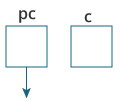Here, a pointer pc and a normal variable c, both of type `int`, is created.
Since pc and c are not initialized at initially, pointer pc points to either no address or a random address. And, variable c has an address but contains random garbage value.

2. `c = 22;`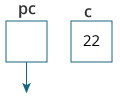This assigns 22 to the variable c. That is, 22 is stored in the memory location of variable c.

3. `pc = &c;`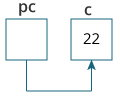This assigns the address of variable c to the pointer pc.

4. `c = 11;`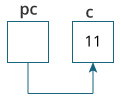This assigns 11 to variable c.

5. `*pc = 2;`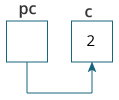This change the value at the memory location pointed by the pointer pc to 2.

### Common mistakes when working with pointers

Suppose, you want pointer pc to point to the address of c. Then,

``````int c, *pc;

// pc is address but c is not
pc = c;  // Error

// &c is address but *pc is not
*pc = &c;  // Error

// both &c and pc are addresses
pc = &c;  // Not an error

// both c and *pc are values
*pc = c;  // Not an error``````

Here's an example of pointer syntax beginners often find confusing.

``````#include <stdio.h>
int main() {
int c = 5;
int *p = &c;

printf("%d", *p);  // 5
return 0;
}``````

Why didn't we get an error when using `int *p = &c;`?

It's because

``int *p = &c;``

is equivalent to

``````int *p:
p = &c;``````

In both cases, we are creating a pointer `p` (not `*p`) and assigning `&c` to it.

To avoid this confusion, we can use the statement like this:

``int* p = &c;``

Now you know what pointers are, you will learn how pointers are related to arrays in the next tutorial.